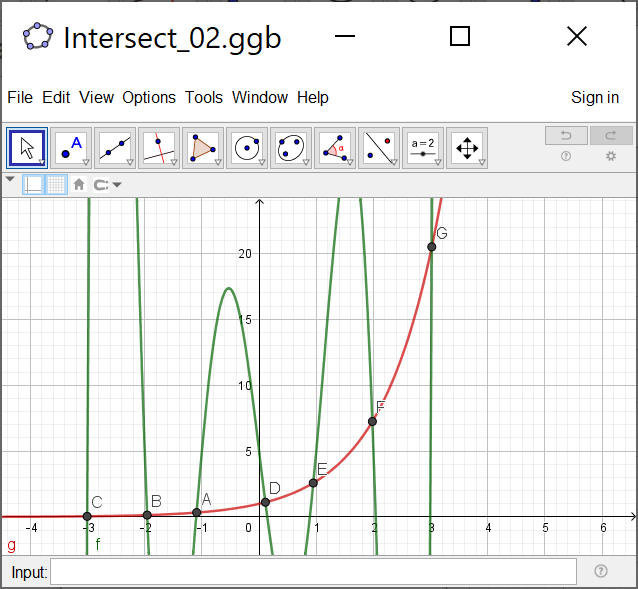# Intersect(function,function,x,y)

EKluge shared this question 2 years ago

Hello,

I am trying out the Interscet command for arbitary functions ,like

g(x) = exp(x) with

f(x) = (x^2-1) (x^2-4) (x^2-9) +5

f(x) = (x^2-1) (x^2-4) (x^2-9) x +5

the command doesn't work for arbitary complicated functions like the second f(x).

There are not all Intersections found.

Do anyone have an idea, why not?

I tried it on Geogebra Classic 5.0 and 6.0 as well.

Sincerely yours1

Gib mal mehr Nachkommastellen...

Erweitere den Suchbereich

ggf. numerisch NSolutions()1

`Intersect(f, g, -4, 4)`